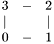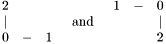# imaging::Image2Grid< fem_types > Class Template Reference [Finite Element Module]

Constructs FE grids from multi-dimensional images and provides conversion functions between grid and image. More...

#include <Image2Grid.hpp>

List of all members.

## Public Member Functions

Image2Grid (const ublas::fixed_vector< size_t, fem_types::data_dimension > &dimensions)
void construct_grid (Grid< fem_types > &grid) const
template<class vector_image_accessor_t>
void construct_grid (Grid< fem_types > &grid, const vector_image_accessor_t &displacements) const
void stiffness_matrix_prototype (ublas::compressed_matrix< float_t > &stiffness_matrix, size_t system_size=1) const
template<class float_accessor_t>
void image2vector (const float_accessor_t &image, ublas::vector< float_t > &vector) const
template<class float_accessor_t>
void vector2image (const ublas::vector< float_t > &vector, float_accessor_t &image) const
template<class vector_image_accessor_t>
void vector2vector_image (const ublas::vector< float_t > &vector, vector_image_accessor_t &vector_image) const
template<class float_accessor_t>
void image2boundary_vector (const float_accessor_t &image, ublas::mapped_vector< float_t > &vector) const

## Detailed Description

### template<class fem_types> class imaging::Image2Grid< fem_types >

Constructs FE grids from multi-dimensional images and provides conversion functions between grid and image.

This class constructs a FE grid for a given image which reflects the geomotry of the image data. The type of the grid is determined by the template parameter fem_types. Its dimension (fem_types::data_dimension) must be the same as the dimension of input image data. Additionally the class provides functions to convert (multi-dimensional) image data to the 1-dimensional vector representation necessary for FE computations and back again.

Note that all the functions in this class are implemented for specific fem_types classes only (mainly fem_2d_square_types and fem_2d_triangle_types). For fem_2d_square_types the structure of a pixel is the following:For fem_2d_triangle_types the structure of a pixel is the following:## Constructor & Destructor Documentation

template<class fem_types>
 imaging::Image2Grid< fem_types >::Image2Grid ( const ublas::fixed_vector< size_t, fem_types::data_dimension > & dimensions )  [inline]

Constructs an Image2Grid objects which creates the grid determined by dimensions or converts data from and to it.

## Member Function Documentation

template<class fem_types>
 void imaging::Image2Grid< fem_types >::construct_grid ( Grid< fem_types > & grid ) const [inline]

Sets the geometry of grid to the dimensions specified in the constructor of the Image2Grid object.

template<class fem_types>
template<class vector_image_accessor_t>
 void imaging::Image2Grid< fem_types >::construct_grid ( Grid< fem_types > & grid, const vector_image_accessor_t & displacements ) const [inline]

Sets the geometry of grid to the dimensions specified in the constructor of the Image2Grid object. Each node of the grid is displaced by the displacement vectors in displacements.

template<class fem_types>
 void imaging::Image2Grid< fem_types >::stiffness_matrix_prototype ( ublas::compressed_matrix< float_t > & stiffness_matrix, size_t system_size = 1 ) const [inline]

Resizes stiffness_matrix to the correct size for grid and prefilled with values at matrix position where non-zero entries are expected (this depends on the geometry of the grid). In case the PDE to be solved is not scalar but a system of equation, the user has to pass the number of equations (system_size) to ensure that stiffness_matrix is sized correctly.

template<class fem_types>
template<class float_accessor_t>
 void imaging::Image2Grid< fem_types >::image2vector ( const float_accessor_t & image, ublas::vector< float_t > & vector ) const [inline]

Converts image to vector such that the indices of the values in vector conform with the node indices of the grid constructed by this Image2Grid object.

Parameters:
 [in] image An Image or ImageAccessorInterface object which has scalar (grayscale) values. The dimensions of image must match the dimensions of the grid, otherwise an Exception is thrown. [out] vector Is automatically resized to the number of nodes in the grid.

template<class fem_types>
template<class float_accessor_t>
 void imaging::Image2Grid< fem_types >::vector2image ( const ublas::vector< float_t > & vector, float_accessor_t & image ) const [inline]

Converts vector to image such that the indices of the values in vector conform with the node indices of the grid constructed by this Image2Grid object.

Parameters:
 [in] vector Its length must match the number of nodes on the grid, otherwise an Exception is thrown. [out] image An Image or ImageAccessorInterface object which has scalar (grayscale) values. The dimensions of image must match the dimensions of the grid, otherwise an Exception is thrown.

template<class fem_types>
template<class vector_image_accessor_t>
 void imaging::Image2Grid< fem_types >::vector2vector_image ( const ublas::vector< float_t > & vector, vector_image_accessor_t & vector_image ) const [inline]

Converts vector to vector_image such that the indices of the values in vector conform with the node indices of the grid constructed by this Image2Grid object.

Parameters:
 [in] vector Its length must match the number of nodes on the grid times the dimension of the values of vector_image, otherwise an Exception is thrown. [out] vector_image An Image or ImageAccessorInterface object which has vector values. The dimensions of vector_image must match the dimensions of the grid, otherwise an Exception is thrown.

template<class fem_types>
template<class float_accessor_t>
 void imaging::Image2Grid< fem_types >::image2boundary_vector ( const float_accessor_t & image, ublas::mapped_vector< float_t > & vector ) const [inline]

Converts image to vector such that the indices of the values in vector conform with the node indices of the grid constructed by this Image2Grid object. In contrast to image2vector() only the image values at the boundary are copied.

Parameters:
 [in] image An Image or ImageAccessorInterface object which has scalar (grayscale) values. The dimensions of image must match the dimensions of the grid, otherwise an Exception is thrown. [out] vector Is automatically resized to the number of nodes in the grid.

The documentation for this class was generated from the following file:

Generated on Tue Feb 10 10:01:31 2009 for imaging2 by1.5.5